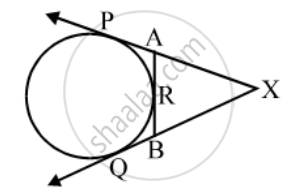Share

Books Shortlist

In fig. XP and XQ are tangents from X to the circle with centre O. R is a point on the circle. Prove that, XA + AR = XB + BR. - CBSE Class 10 - Mathematics

ConceptCircles Examples and Solutions

Question

In fig. XP and XQ are tangents from X to the circle with centre O. R is a point on the circle. Prove that, XA + AR = XB + BR.

Solution

Since lengths of tangents from an exterior point to a circle are equal.∴ XP = XQ …. (i) [From X]

AP = AR …. (ii) [From A]

BQ = BR …. (iii) [From B]

Now, XP = XQ

⇒ XA + AP = XB + BQ

⇒ XA + AR = XB + BR [Using equations (i) and (ii)]

Is there an error in this question or solution?

Video TutorialsVIEW ALL 

Solution In fig. XP and XQ are tangents from X to the circle with centre O. R is a point on the circle. Prove that, XA + AR = XB + BR. Concept: Circles Examples and Solutions.
S